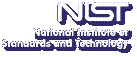Up# Cryptanalysis of RSA Variants and Implicit Factorization

Santanu Sarkar
National Institute of Standards and Technology

Tuesday, August 20, 2013 15:00-16:00,
Building 225, Room B111
Gaithersburg
Tuesday, August 20, 2013 13:00-14:00,
Room 1-4058
Boulder

Abstract:

The famous RSA public key cryptosystem is possibly the most studied topic in cryptology research. Apart from the basic RSA proposal there are several variants of it for efficiency and security purposes. In this talk we will first discuss three RSA variants Dual RSA, Common Prime RSA and Prime Power RSA.

Dual RSA have been proposed by Sun et al. (IEEE-IT, August 2007). We here concentrate on the Dual CRT-RSA scheme and present certain range of parameters for which it is insecure. Next we consider the Common Prime RSA as proposed by Wiener (IEEE-IT, 1990). We present new range of parameters in Common Prime RSA for which it is not secure. After that we consider an RSA variant with Modulus $N = p^rq$. This variant is known as Prime Power RSA. In PKC 2004, May proved when decryption exponent $d \lt N^{\frac{r}{(r+1)^2}}$ or $d \lt N^{\left( \frac{r-1}{r+1} \right) ^2}$, one can factor $N$ in polynomial time. We improve this bound when $r \le 5$.

Then we study Implicit Factorization. Implicit Factorization was introduced by May and Ritzenhofen in PKC 2009 and studied under the assumption that some of Least Significant Bits (LSBs) of certain primes are the same. Our strategy can be applied to the implicit factorization problem in a general framework considering the equality of (i) Most Signifiant Bits (MSBs), (ii) Least Significant Bits (LSBs) and (iii) MSBs and LSBs together.

Finally we cryptanalysis CRT-RSA with low Hamming weight decryption exponents. We show that the CRT-RSA schemes presented by Lim and Lee (SAC 1996) and Galbraith, Heneghan and McKee (ACISP 2005) with low weight decryption exponents can be broken in a few minutes in certain cases.

Speaker Bio: Santanu Sarkar is a guest researcher at National Institute of Standards and Technology. He is also an assistant professor in Chennai Mathematical Institute, India. He received Ph.D degree in Mathematics from Indian Statistical Institute, India, in 2011. His main subject interest is cryptology and number theory.

Presentation Slides: PDF

Contact: R. N. Kacker

Note: Visitors from outside NIST must contact Cathy Graham; (301) 975-3800; at least 24 hours in advance.